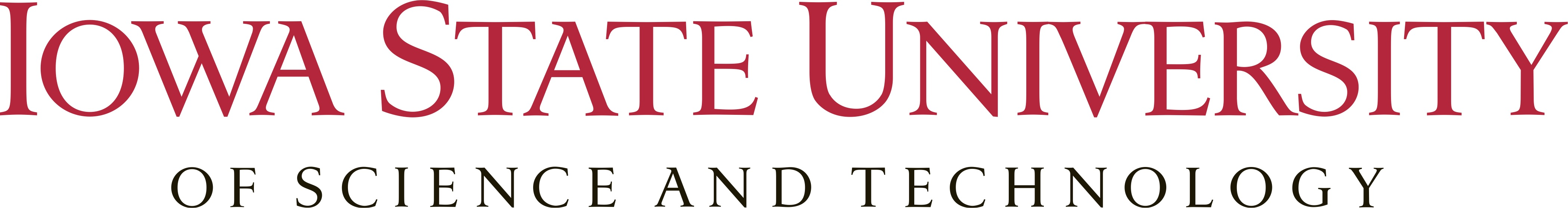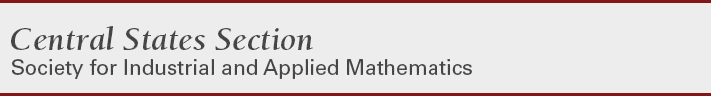### MiniSymposium-14: Nonlocal Models: Analysis Aspects

Abstract: Nonlocal theories have been successfully employed in many settings, such as fracture modeling, image processing, biology, sand pile formation, and more. Nonlocal models use integral operators which take into account the cumulative behavior in the neighborhood of a point, and thus they allow solutions or domains to be discontinuous. On the other hand, nonlocal models based on integro-differential equations have fundamentally different mathematical structure and properties compared to their local PDE-based counterparts, requiring new and novel analytical and numerical techniques to analyze and utilize them. In this minisymposium we will focus on recent advances in nonlocal theories, including theoretical results (well-posedness, regularity, and asymptotic behavior of solutions), numerical methods and analysis (such as convergence and conditioning), and practical application (modeling, simulation, engineering analysis) related to nonlocal theories that have appeared in fracture modeling (peridynamics), nonlocal diffusion, biology, image processing, and more.

Organizers:

Saturday, October 19th, 2019 at 10:20 - 11:40 (268 Carver Hall)
10:20 - 10:40 Pablo Raúl Stinga,
Iowa State University
The Obstacle Problem for a Fractional Monge-Ampère Equation

We study the obstacle problem for a degenerate fractional Monge-Ampère equation. This nonlocal operator was introduced by Caffarelli and Charro. We show the existence of a unique, globally Lipschitz and semiconcave classical solution, at which the fractional Monge-Ampère equation becomes locally uniformly elliptic. The uniform ellipticity is used to deduce local regularity of the solution and the free boundary. This is joint work with Yash Jhaveri (IAS).

10:40 - 11:00 Mikil Foss,
Poincare Inequalities for Nonhomogeneous Nonlocal Operators

Convolution operators with integrable kernels have become an important component for nonlocal models of phenomenon with discontinuities, such as fracture and tearing. For these models, existence and stability results often rely on coercivity properties of the operator in an appropriate space. I will present some new Poincare type inequalities for nonhomogeneous operators that provide coercivity in Lebesgue spaces.

11:00 - 11:20 Simon Bortz,
University of Washington - Seattle
Some Soft Tools for PDE Regularity

Complex analysis and functional analysis come together in some very interesting and powerful ways. Perhaps the most useful fusion of these fields is the method of complex interpolation, which will be the primary tool in this talk. We will show how to exploit features of complex interpolation in a variety of situations, (local and non-local elliptic and parabolic equations) to show improved integrability and/or differentiability properties of solutions to differential equations. Time permitting we will discuss some future directions.

11:20 - 11:40 Hatim Khudhair,
Missouri University of Science and Technology
Turing Patterns in the Schnakenberg Equations: From Normal to Anomalous Diffusion

In recent years, anomalous diffusion has been observed in many biological experiments. Instead of classical Laplace operator, the anomalous diffusion is described by the fractional Laplacian, leading to nonlocal models. In this talk, we will discuss the Turing patterns of the Schnakenberg equation and compare the pattern selection under normal and anomalous diffusion. Our analysis shows that the wave number of the Turing instability increases with the exponent of the fractional Laplacian. The interplay of the nonlinearity and long-range diffusion are studied numerically, and especially comparisons are provided to understand the nonlocal effects of the fractional Laplacian.

Saturday, October 19th, 2019 at 14:40 - 16:00 (268 Carver Hall)
14:40 - 15:00 Cory Wright,
Lehigh University
Nonlocal Trace Theorems and Their Applications

The classical trace theory demonstrates that the trace operator on continuous functions can be extended continuously as a map on sobolev spaces $$H^1$$ to $$H^{1/2}$$. This theory has a myriad of useful applications throughout different aspects of analysis. It is then natural to study similar theories for the nonlocal framework, more specifically peridynamics. We will show two different versions of a nonlocal trace theorem involving peridynamic kernels. We define a nonlocal energy space motivated by a nonlocal gradient operator and use this as a basis to create a trace theorem involving $$L^2$$. Viewing this energy space as a nonlocal analogue to $$H^1$$ also motivates a nonlocal trace theorem incorporating a nonlocal version of the space $$H^{1/2}$$ as in the classical trace theory. We then will demonstrate some useful applications of these theorems to different problems in nonlocal analysis.

15:00 - 15:20 Mary Vaughan,
Iowa State University
Fractional Derivatives in One-sided Weighted Sobolev Spaces

Fractional derivatives can be defined in the most general way in the distributional sense. We start from this point and then show that the Marchaud pointwise formulas for these operators indeed hold for functions in more general classes. The classes we consider are weighted Sobolev spaces with one-sided Sawyer weights. These classes capture the one-sided nature of fractional derivatives. The pointwise and norm limits as the orders of the derivatives converge to an integer are also analyzed.

15:20 - 15:40 Animesh Biswas,
Iowa State University
Regularity Theory for Nonlocal Space-time Master Equations

We analyze recent novel regularity theory for fractional power of parabolic operators in divergence form. These equations are fundamental in continuous time random walk models and appear as generalized Master equation. These equations are non-local in nature and were studied by Luis Caffarelli and Luis Silvestre. We developed a parabolic method of semigroups that allows us to prove a local extension problem. As a consequence we obtain interior and boundary Harnack inequalities and sharp interior and global parabolic Schauder estimates. For the latter, we also prove a characterization of the correct intermediate parabolic Hölder spaces in the spirit of Sergio Campanato. This is joint work with Marta de León-Contreras (Universidad Autonoma de Madrid, Spain) and Pablo Raúl Stinga (Iowa State University).

15:40 - 16:00 Evan Camrud,
Iowa State University
Fractional Operators via Analytic Interpolation of Integer Powers

Although the study of functional calculus has already established necessary and sufficient conditions for operators to be fractionalized, this paper aims to use our well-conceived notion of integer powers of operators to construct non-integer powers of operators. In doing so, we not only provide a more intuitive understanding of fractional theories, but also provide a framework for producing new fractional theories. Such interpolations allow one to approximate fractional powers by finite sums of integer powers of operators, and thus may find much use in numerical analysis of fractional PDEs and the time-frequency analysis of the fractional Fourier transform. Further, these results provide an interpolation procedure for the Riemann zeta function.

Coordinated by
Iowa State University